# iOS CoreAnimation专题——实战篇（四）基于拖动手势的视图3D旋转效果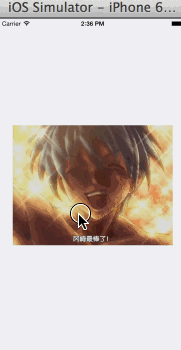# 矩阵变换

## 基向量

a=(x,0),b=(0,y)v=a+b a → = ( x , 0 ) , b → = ( 0 , y ) ， 有 v → = a → + b →

a=xi,b=yj a → = x i → , b → = y j →

v=a+b=xi+yj v → = a → + b → = x i → + y j →

v=ni+mj=(na,nb)+(mc,md)=(na+mc,nb+md)=(x,y) v → = n i → + m j → = ( n a , n b ) + ( m c , m d ) = ( n a + m c , n b + m d ) = ( x , y )

{xy=na+mc=nb+md { x = n a + m c y = n b + m d

n=dxcyadbc,m=aybxadbc n = d x − c y a d − b c , m = a y − b x a d − b c

v=ni+mj=dxcyadbci+aybxadbcj v → = n i → + m j → = d x − c y a d − b c i → + a y − b x a d − b c j →

## 线性变换

vector f(vector v) {
vector x = v进行某些计算后的结果
return x;
}

x=f(v) x → = f ( v → )

ok，这“某些条件”是哪些条件啊？按照书上对线性的定义，函数需要满足：

$f\left(\stackrel{\to }{v}+\stackrel{\to }{u}\right)=f\left(\stackrel{\to }{v}\right)+f\left(\stackrel{\to }{u}\right)$

f(cv)=cf(v) f ( c v → ) = c f ( v → )

i=(1,0),j=(0,1) i → = ( 1 , 0 ) , j → = ( 0 , 1 )

L(v)=L(xi+yj)=L(xi)+L(yj)=xL(i)+yL(j) L ( v → ) = L ( x i → + y j → ) = L ( x i → ) + L ( y j → ) = x L ( i → ) + y L ( j → )

L(v)=xL(i)+yL(j) L ( v → ) = x L ( i → ) + y L ( j → )

v=xi+yj v → = x i → + y j →

u=L(v),k=L(i),l=L(j) u → = L ( v → ) , k → = L ( i → ) , l → = L ( j → )

u=xk+yl(1) (1) u → = x k → + y l →

v=xi+yj(2) (2) v → = x i → + y j →

L(v)=xk+yl=(x,y)(kl)(3) (3) L ( v → ) = x k → + y l → = ( x , y ) ( k → l → )

L(v)=xk+yl=(x,y)(kl)=(x,y)(acbd)=(x,y)At=vAt L ( v → ) = x k → + y l → = ( x , y ) ( k → l → ) = ( x , y ) ( a b c d ) = ( x , y ) A t = v → A t

L(v)=vAtAt=(kl)=(acbd) L ( v → ) = v → A t ， 其 中 A t = ( k → l → ) = ( a b c d )

vector linear_transformation(vector v) {
// 找到该变换对应的矩阵
matrix At = (a,b,c,d);
// 输入向量左乘矩阵A
vector u = vAt;

return u;
}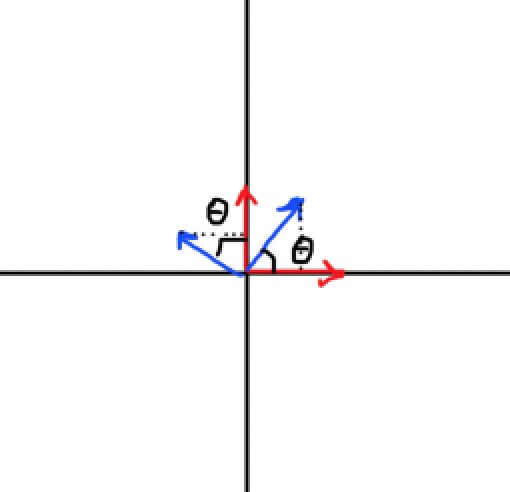k=(cosθ,sinθ)l=(sinθ,cosθ) k → = ( cos ⁡ θ , sin ⁡ θ ) ， l → = ( − sin ⁡ θ , cos ⁡ θ )

A=(cosθsinθsinθcosθ) A = ( cos ⁡ θ sin ⁡ θ − sin ⁡ θ cos ⁡ θ )

u=L(v)=vAt=(x,y)(cosθsinθsinθcosθ)=(xcosθysinθ,xsinθ+ycosθ) u → = L ( v → ) = v → A t = ( x , y ) ( cos ⁡ θ sin ⁡ θ − sin ⁡ θ cos ⁡ θ ) = ( x cos ⁡ θ − y sin ⁡ θ , x sin ⁡ θ + y cos ⁡ θ )

## 线性变换的复合

w=uAscale=(vArotate)Ascale w → = u → A s c a l e = ( v → A r o t a t e ) A s c a l e

w=(vArotate)Ascale=v(ArotateAscale)=vAt w → = ( v → A r o t a t e ) A s c a l e = v → ( A r o t a t e A s c a l e ) = v → A t

At=ArotateAscale A t = A r o t a t e A s c a l e

# 齐次坐标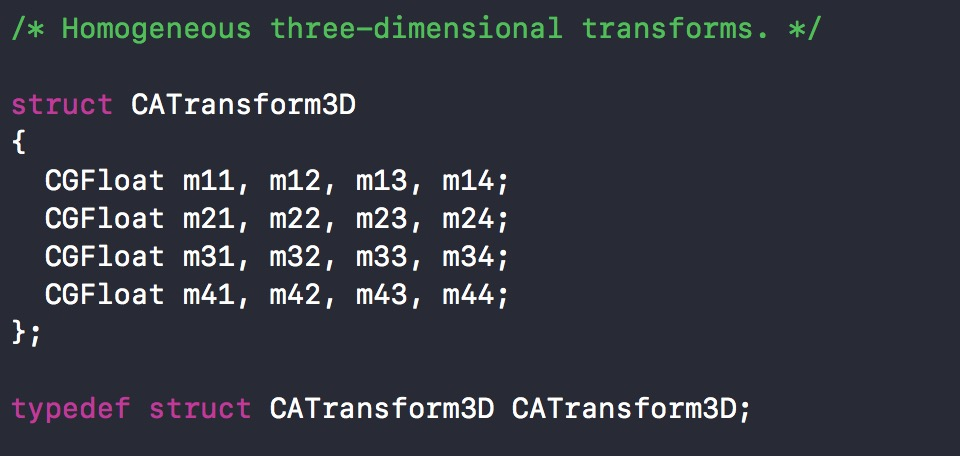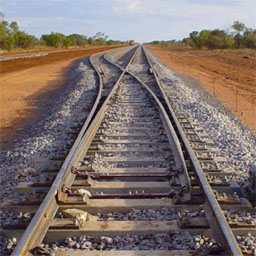## 齐次坐标下的点和向量的区别

v=xi+yj(1) (1) v → = x i → + y j →

PO=v P − O = v →

$\begin{array}{}\text{(2)}& P\left(x,y\right)=x\stackrel{\to }{i}+y\stackrel{\to }{j}+O\end{array}$

v=(x,y)(ij)=(x,y,0)ijO v → = ( x , y ) ( i → j → ) = ( x , y , 0 ) ( i → j → O )

P=(x,y,1)ijO P = ( x , y , 1 ) ( i → j → O )

## 齐次坐标下的平移变换

(x,y)At=(x+a,y+b) ( x , y ) A t = ( x + a , y + b )

(x,y,1)At=(x+a,y+b,1) ( x , y , 1 ) A t = ( x + a , y + b , 1 )

Arotate=cossin0sincos0001,Ascale=sx000sy0001 A r o t a t e = ( c o s s i n 0 − s i n c o s 0 0 0 1 ) , A s c a l e = ( s x 0 0 0 s y 0 0 0 1 )

(x,y,0)At=(x+a,y+b,0) ( x , y , 0 ) A t = ( x + a , y + b , 0 )

f(x,y)=(x+a,y+b) f ( x , y ) = ( x + a , y + b )

f(U+V)=f(ux+vx,uy+vy)=(ux+vx+a,uy+vy+b) f ( U + V ) = f ( u x + v x , u y + v y ) = ( u x + v x + a , u y + v y + b )

f(U)+f(V)=f(ux,uy)+f(vx,vy)=(ux+a,uy+b)+(vx+a,vy+b)=(ux+vx+2a,uy+vy+2b) f ( U ) + f ( V ) = f ( u x , u y ) + f ( v x , v y ) = ( u x + a , u y + b ) + ( v x + a , v y + b ) = ( u x + v x + 2 a , u y + v y + 2 b )

f(U+V)f(U)+f(V) f ( U + V ) ≠ f ( U ) + f ( V )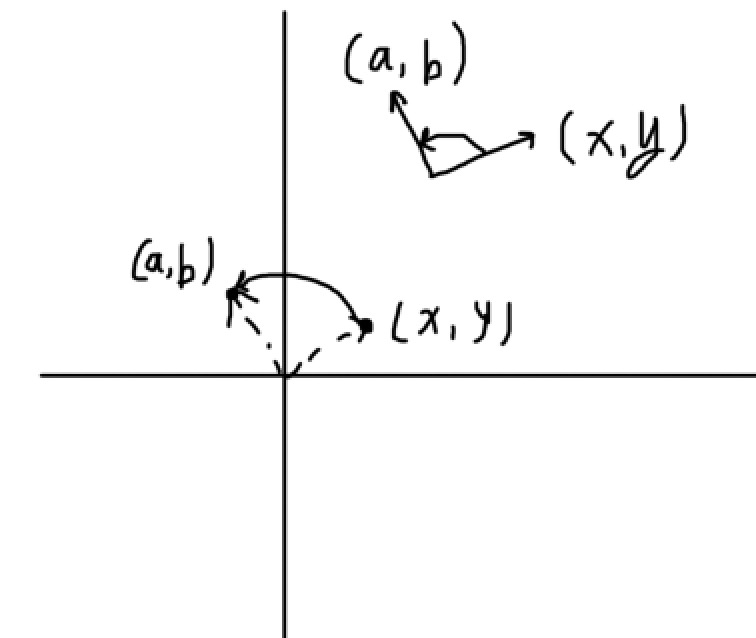## 齐次坐标下的平行线相交问题

{Ax+By+C=0Ax+By+D=0 { A x + B y + C = 0 A x + B y + D = 0

Axk+Byk+C=0Axk+Byk+D=0 { A x ′ k + B y ′ k + C = 0 A x ′ k + B y ′ k + D = 0

{Ax+By+Ck=0Ax+By+Dk=0 { A x ′ + B y ′ + C k = 0 A x ′ + B y ′ + D k = 0

# CATransform3D

1. 图像是由像素点构成的，要实现图像的各种变形变换，需要一个变换函数，将一个点作为输入参数，输出变换后的点。把构成该图像的所有像素点都拿去调用这个函数，就能实现图像的变形了；
2. 在线性代数中有一种变换叫线性变换，它接收一个向量作为输入参数，输出变形后的向量，比如一个逆时针旋转90°的线性变换，接收任何一个向量，输出的向量就是原向量逆时针旋转90°后的向量；
3. 线性变换的过程实际上就是输入向量乘以某个变换矩阵。我们需要实现的三种基本变换：平移、缩放、旋转中，缩放和旋转是线性变换（满足可加性和一阶齐次），由于矩阵乘法拥有结合律，所以多个线性变换可以通过它们的变换矩阵相乘复合成一个变换矩阵，比如我们可以用缩放矩阵乘以旋转矩阵，得到的结果就是一个描述先缩放再旋转的变换矩阵，输入向量乘以这个矩阵，输出的向量就是先缩放后旋转的结果；
4. 对于平移变换，为了让它也能通过矩阵乘法进行变换的复合，我们发现只有在齐次坐标下才能找到这样一个矩阵，而为了能让平移变换也加入旋转和缩放的复合运算中，旋转和缩放也应该在齐次坐标下来表示，这样它们才能相乘。

## 基于CATransform3D的变换矩阵

// 让一个layer在x轴方向拉伸2倍，在y轴方向拉伸3倍，在z轴方向拉伸1倍。
// 当然一般的layer是没有厚度的概念的，所以z轴的拉伸对layer而言就是没有意义的
layer.transform = CATransform3DMakeScale(2, 3, 1);

/* Returns a transform that scales by (sx, sy, sz)':
* t' = [sx 0 0 0; 0 sy 0 0; 0 0 sz 0; 0 0 0 1]. */

CA_EXTERN CATransform3D CATransform3DMakeScale (CGFloat sx, CGFloat sy,
CGFloat sz)
CA_AVAILABLE_STARTING (10.5, 2.0, 9.0, 2.0);

t=sx0000sy0000sz00001 t ′ = ( s x 0 0 0 0 s y 0 0 0 0 s z 0 0 0 0 1 )

xi=(1,0,0),yj=(0,1,0),zk=(0,0,1) x 轴 的 基 向 量 i → = ( 1 , 0 , 0 ) , y 轴 的 基 向 量 j → = ( 0 , 1 , 0 ) , z 轴 的 基 向 量 k → = ( 0 , 0 , 1 ) $x轴的基向量\vec{i} = (1,0,0),y轴的基向量\vec{j} = (0,1,0),z轴的基向量\vec{k} = (0,0,1)$

si=(sx,0,0),sj=(0,sy,0),sk=(0,0,sz) s i → = ( s x , 0 , 0 ) , s j → = ( 0 , s y , 0 ) , s k → = ( 0 , 0 , s z )

t=sx000sy000sz t = ( s x 0 0 0 s y 0 0 0 s z )

/* Returns a transform that translates by '(tx, ty, tz)':
* t' =  [1 0 0 0; 0 1 0 0; 0 0 1 0; tx ty tz 1]. */

CA_EXTERN CATransform3D CATransform3DMakeTranslation (CGFloat tx,
CGFloat ty, CGFloat tz)
CA_AVAILABLE_STARTING (10.5, 2.0, 9.0, 2.0);

/* Returns a transform that scales by (sx, sy, sz)':
* t' = [sx 0 0 0; 0 sy 0 0; 0 0 sz 0; 0 0 0 1]. */

CA_EXTERN CATransform3D CATransform3DMakeScale (CGFloat sx, CGFloat sy,
CGFloat sz)
CA_AVAILABLE_STARTING (10.5, 2.0, 9.0, 2.0);

/* Returns a transform that rotates by 'angle' radians about the vector
* '(x, y, z)'. If the vector has length zero the identity transform is
* returned. */

CA_EXTERN CATransform3D CATransform3DMakeRotation (CGFloat angle, CGFloat x,
CGFloat y, CGFloat z)
CA_AVAILABLE_STARTING (10.5, 2.0, 9.0, 2.0);

/* Translate 't' by '(tx, ty, tz)' and return the result:
* t' = translate(tx, ty, tz) * t. */

CA_EXTERN CATransform3D CATransform3DTranslate (CATransform3D t, CGFloat tx,
CGFloat ty, CGFloat tz)
CA_AVAILABLE_STARTING (10.5, 2.0, 9.0, 2.0);

/* Scale 't' by '(sx, sy, sz)' and return the result:
* t' = scale(sx, sy, sz) * t. */

CA_EXTERN CATransform3D CATransform3DScale (CATransform3D t, CGFloat sx,
CGFloat sy, CGFloat sz)
CA_AVAILABLE_STARTING (10.5, 2.0, 9.0, 2.0);

/* Rotate 't' by 'angle' radians about the vector '(x, y, z)' and return
* the result. If the vector has zero length the behavior is undefined:
* t' = rotation(angle, x, y, z) * t. */

CA_EXTERN CATransform3D CATransform3DRotate (CATransform3D t, CGFloat angle,
CGFloat x, CGFloat y, CGFloat z)
CA_AVAILABLE_STARTING (10.5, 2.0, 9.0, 2.0);

/* Concatenate 'b' to 'a' and return the result: t' = a * b. */

CA_EXTERN CATransform3D CATransform3DConcat (CATransform3D a, CATransform3D b)
CA_AVAILABLE_STARTING (10.5, 2.0, 9.0, 2.0);


// 这是一个数学公式而不是赋值语句
CATransform3DScale(t,sx,sy,sz) = CATransform3DConcat(t,CATransform3DMakeScale(sx,sy,sz))


/* The identity transform: [1 0 0 0; 0 1 0 0; 0 0 1 0; 0 0 0 1]. */

CA_EXTERN const CATransform3D CATransform3DIdentity

## 3D旋转变换

/* Returns a transform that rotates by 'angle' radians about the vector
* '(x, y, z)'. If the vector has length zero the identity transform is
* returned. */

CA_EXTERN CATransform3D CATransform3DMakeRotation (CGFloat angle, CGFloat x,
CGFloat y, CGFloat z)
CA_AVAILABLE_STARTING (10.5, 2.0, 9.0, 2.0);

- (void)touchesEnded:(NSSet<UITouch *> *)touches withEvent:(UIEvent *)event
{
CALayer * layer = [CALayer layer];
layer.frame = CGRectMake(0, 0, 320, 240);
layer.position = self.view.center;
layer.contents = (__bridge id)[UIImage imageNamed:@"1.jpg"].CGImage;

// 为了让效果更直观，我们写个动画出来看，让layer绕着x轴旋转的动画
CABasicAnimation * animation = [CABasicAnimation animation];
animation.keyPath = @"transform";
animation.duration = 5;
animation.removedOnCompletion = NO;
animation.fillMode = kCAFillModeForwards;
// 绕x轴旋转π/4

CATransform3D transform = CATransform3DMakeRotation(M_PI/4, 1, 0, 0);

animation.toValue = [NSValue valueWithCATransform3D:transform];
}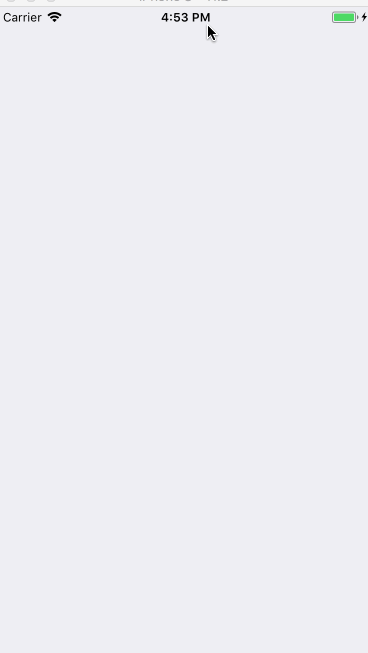## 带透视效果的CATransform3D旋转

- (void)touchesEnded:(NSSet<UITouch *> *)touches withEvent:(UIEvent *)event
{
CALayer * layer = [CALayer layer];
layer.frame = CGRectMake(0, 0, 320, 240);
layer.position = self.view.center;
layer.contents = (__bridge id)[UIImage imageNamed:@"1.jpg"].CGImage;

CABasicAnimation * animation = [CABasicAnimation animation];
animation.keyPath = @"transform";
animation.duration = 5;
animation.removedOnCompletion = NO;
animation.fillMode = kCAFillModeForwards;

// 设置透视变换矩阵
CATransform3D perspectiveTransform = CATransform3DIdentity;
perspectiveTransform.m34 = -1.f/700;
// 将透视变换复合到旋转变换中
// 绕x轴旋转π/4
CATransform3D transform = CATransform3DRotate(perspectiveTransform, M_PI/4, 1, 0, 0);

animation.toValue = [NSValue valueWithCATransform3D:transform];
}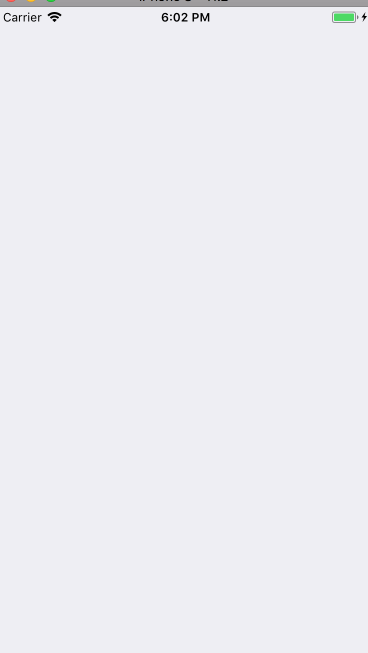# 基于Pan手势的3D旋转控制

## 总体实现思路

1. 这是一个3D旋转效果；
2. 这个3D旋转效果是由pan手势控制的；
3. 旋转的方向就是手指移动的方向。

CATransform3D CATransform3DRotate (CATransform3D t, CGFloat angle,
CGFloat x, CGFloat y, CGFloat z)

## 通过向量计算当前手指的移动方向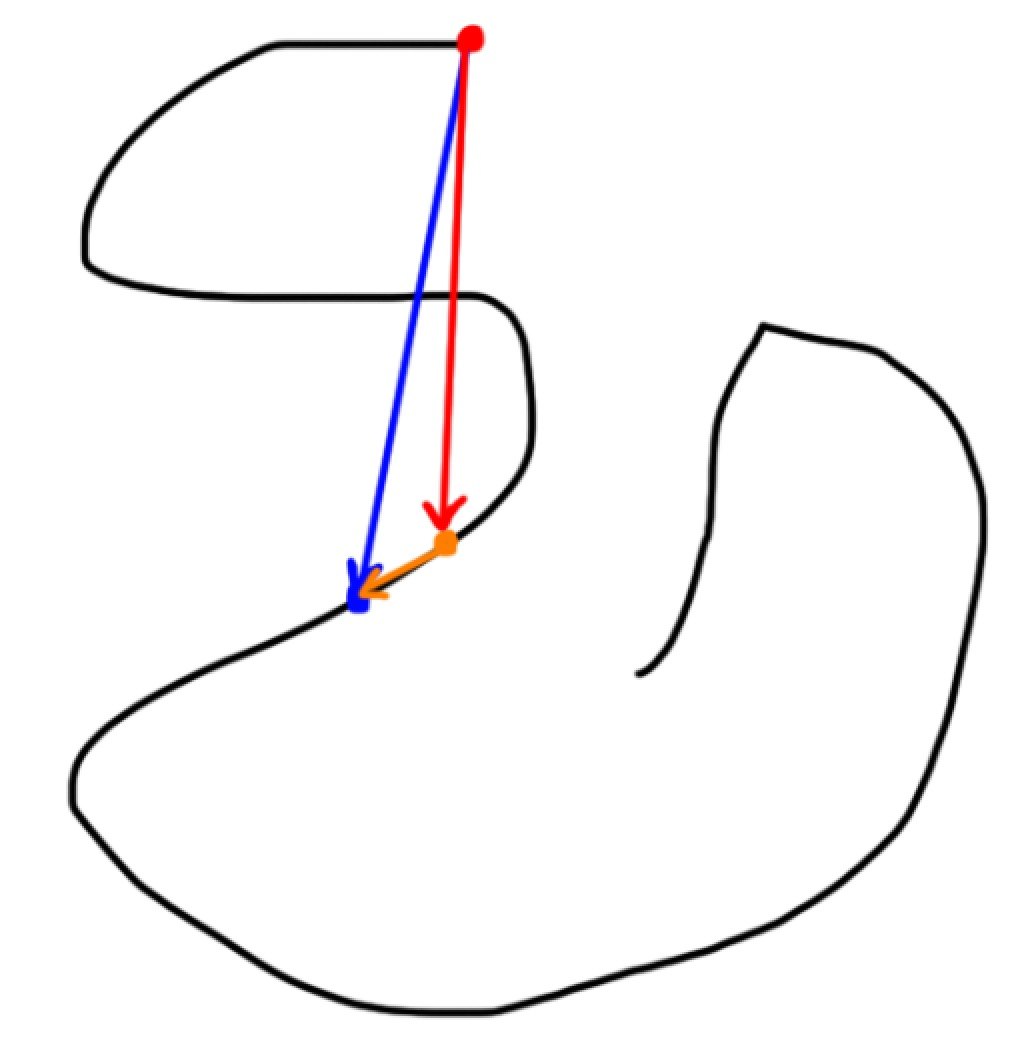v+v=v=>v=vv v 红 → + v 橙 → = v 蓝 → => v 橙 → = v 蓝 → − v 红 →

- (void)onPanGesture:(UIPanGestureRecognizer *)sender
{
if (sender.state == UIGestureRecognizerStateBegan) {

} else if (sender.state == UIGestureRecognizerStateChanged) {

// 获取当前手指的位移（translation）
CGPoint panTranslation = [sender translationInView:sender.view];
// 我们要的向量是手指上次所在的点到这次所在的点连成的一个向量，这是你这次手指滑动的方向，传给transform3DRotate函数的向量是垂直于这个向量的向量。而我们已知的只有这个transition，也就是手指最开始的点到手指当前点连成的一个向量（也就是手指的位移，只考虑起始点和结束点）。
// 画出图来就发现，我们要的向量就是当前向量-上一次手指的位移向量（向量减法）

// 通过这个位移生成一个向量，这就是我们当前的位移向量。
DHVector * vector = [[DHVector alloc] initWithCoordinateExpression:panTranslation];

// 用当前的位移向量-上次的位移向量得到我们手指的位移偏移量
DHVector * translateVector = [DHVector aVector:vector substractedByOtherVector:[self lastTranslation]];

// 把这个向量保存起来，下次调用这个方法的时候需要拿到这次的向量，用来做减法
// 下次再调用这个方法的时候的lastTranslation就是这次的位移向量，所以用这次的位移向量覆盖掉lastTranslation（用这次的位移向量给lastTranslation赋值）
[self setLastTranslation:vector];

// 随便计算一下单位旋转角度，也就是每次调用这个方法的时候应该旋转多少度（线性插值）

// 生成旋转向量，也就是要传给CATransform3DRotate函数的向量，它通过translateVector顺时针旋转90度（PI/2）得到
DHVector * rotateVector = [DHVector vectorWithVector:translateVector];

// 把旋转向量传给函数
self.layer.transform = CATransform3DRotate(self.layer.transform, radian, rotateVector.coordinateExpression.x,  rotateVector.coordinateExpression.y, 0);

} else if (sender.state == UIGestureRecognizerStateCancelled || sender.state == UIGestureRecognizerStateEnded) {

}
}


// 手指的最大位移量和当手指达到最大位移量时对应的旋转角度，用来插值计算每次手指移动应该旋转多少度
static const CGFloat maxTranslate_ = 400.f;
static const CGFloat maxRotateRadian_   =   M_PI * 2;

@interface ViewController ()

@property (nonatomic, strong) UIView * transformView;
@property (nonatomic, strong) DHVector * lastTranslation;
@property (nonatomic, assign) CGFloat transformUnit;

@end

@implementation ViewController

self.view.backgroundColor = [UIColor groupTableViewBackgroundColor];

self.transformUnit = 1.5;

}

- (void)setTransform3DWithPanTranslation:(CGPoint)translation
{
// 我们要的向量是手指上次所在的点到这次所在的点连成的一个向量，这是你这次手指滑动的方向，传给transform3DRotate函数的向量是垂直于这个向量的向量。而我们已知的只有这个transition，也就是手指最开始的点到手指当前点连成的一个向量（也就是手指的位移，只考虑起始点和结束点）。
// 画出图来就发现，我们要的向量就是当前向量-上一次手指的位移向量（向量减法）

// 通过这个位移生成一个向量。
DHVector * vector = [[DHVector alloc] initWithCoordinateExpression:translation];

// 用当前的位移向量-上次的位移向量得到我们手指的位移偏移量
DHVector * translateVector = [DHVector aVector:vector substractedByOtherVector:[self lastTranslation]];

// 把这个向量保存起来，下次调用这个方法的时候需要拿到这次的向量，用来做减法
[self setLastTranslation:vector];

// 随便计算一下单位旋转角度，也就是每次调用这个方法的时候应该旋转多少度

// 生成旋转向量，也就是要传给CATransform3DRotate函数的向量，它通过translateVector顺时针旋转90度（PI/2）得到
DHVector * rotateVector = [DHVector vectorWithVector:translateVector];

// 把旋转向量传给函数
self.transformView.layer.transform = CATransform3DRotate(self.transformView.layer.transform, radian, rotateVector.coordinateExpression.x,  rotateVector.coordinateExpression.y, 0);
}

#pragma mark - callback
- (void)onPanGesture:(UIPanGestureRecognizer *)sender
{
if (sender.state == UIGestureRecognizerStateBegan) {

} else if (sender.state == UIGestureRecognizerStateChanged) {

[self setTransform3DWithPanTranslation:[sender translationInView:sender.view]];

} else if (sender.state == UIGestureRecognizerStateCancelled || sender.state == UIGestureRecognizerStateEnded) {

}
}

#pragma mark - getter

- (UIView *)transformView
{
if (!_transformView) {
_transformView = ({

UIView * view = [[UIView alloc] initWithFrame:CGRectMake(0, 0, 320, 240)];
view.transformUnit = 1.5;
view.center = self.view.center;
view.backgroundColor = [UIColor blueColor];
view.layer.contents = (__bridge id)[UIImage imageNamed:@"1.jpg"].CGImage;
[view prepareForTransform3D];
view;

});

}
return _transformView;
}

@end


# 总结

CoreAnimation专题的最后一篇终于结束了，整个实践篇的目的在于让大家通过我们原理篇和技巧篇的内容来解决需求中可能遇到的各种各样的动画难题，所以我在实践篇的写作中大量提及思考的过程，阅读起来可能会比较难啃，比较干涩（毕竟都是干货呢），但是我的想法是让大家读完实践篇后不仅能实现实践篇里面那么几个效果，还能拥有动画实现的基本思路（套路，即各种分解动画的思维方式），结合我们的技巧篇的各种工具，能够见招拆招，遇到什么都不怕，这样才是内力的修炼，而不是只会几个固定的招数。内力修炼的过程是比较漫长而痛苦的，我也是一步一步一个坑一个坑走过来的，希望大家都能有所收获吧！

06-071万+07-271万+
08-036596
09-301673
05-09551
11-201047
03-20431
04-242176
03-096044
09-116971
08-125443
01-294468
12-235639
12-102477
11-227366
06-303583
09-242020